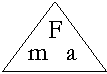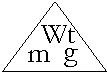# Forces

• A Force is a push or a pull on an object. Forces can:
1. Speed up an object
2. Slow down or stop an object
3. Change the direction of an object
4. Change the shape of an object
• Forces are measured in units called Newtons (N).
• Forces may be balanced (if no movement occurs) or unbalanced (if movement occurs).
• An example of unbalanced forces is the Action and Reaction of a bullet fired from a gun (action), and the following recoil of the gun (reaction).
• Forces may be contact (if objects touch e.g. friction, cohesion and adhesion) or non-contact (if objects do not touch e.g. gravity, static electricity and magnetism).

## The Force Rule

• Force is affected in 2 ways:
1. An object of greater mass has a greater force (e.g. An adult baseballer will hit the ball further than a child)
2. An object with greater acceleration has a greater force (e.g. A faster karate expert can strike with greater force than a slower person)
• Force RuleForce (newtons) = Mass (kilograms) × Acceleration (metre per second-squared) F = m × m/s2 F = m × a F = ma

• Force Rule Example
Question: Daring Darius, the human cannonball whose mass is 100 kg, is accelerated from a cannon at 5 m/s2. What force was used?
 Answer: F = ma = m × a = 100 × 5 = 500 N (newtons)

## Force of Gravity

• Mass is the amount of matter in an object. It is measured in kilograms. The mass of an object remains the same anywhere in the universe.
• Weight is the force of gravity on an object. It is measured in newtons. The weight of an object differs depending on its position in the universe (e.g. A person's weight on the moon with less gravity will be less than that on earth).
• Rule for WeightWeight (newtons) = Mass (kilograms) × Gravitational Acceleration (9.8 m/s2) W = m × g

## Friction

• Friction is a force opposing the movement of one surface over another.
• The Magnitude of the Force of Friction depends on the following factors:
1. The roughness of the surfaces (e.g. stepping on banana peel compared with carpet)
2. The force pushing the surfaces together (e.g. A heavy truck's tyres compared with a bicycle's tyres on the road)
3. Whether the surfaces are moving or stopped

• 3 Types of Friction
1. Static Friction - acting between 2 stationary bodies (e.g. holding a person on a chair)
2. Sliding Friction - acting between surfaces where one is moving (e.g. sliding furniture across the floor, writing with a pencil on paper)
3. Rolling Friction - acting between surfaces of objects where one has a rounded shape (e.g. car tyres on the road, ball bearings); less than sliding friction

• 4 Ways to Reduce Friction
1. Reducing the force pushing both surfaces together
2. Using a lubricant such as oil or water between the surfaces
3. Using ball bearings or rollers between both surfaces
4. Polishing both surfaces